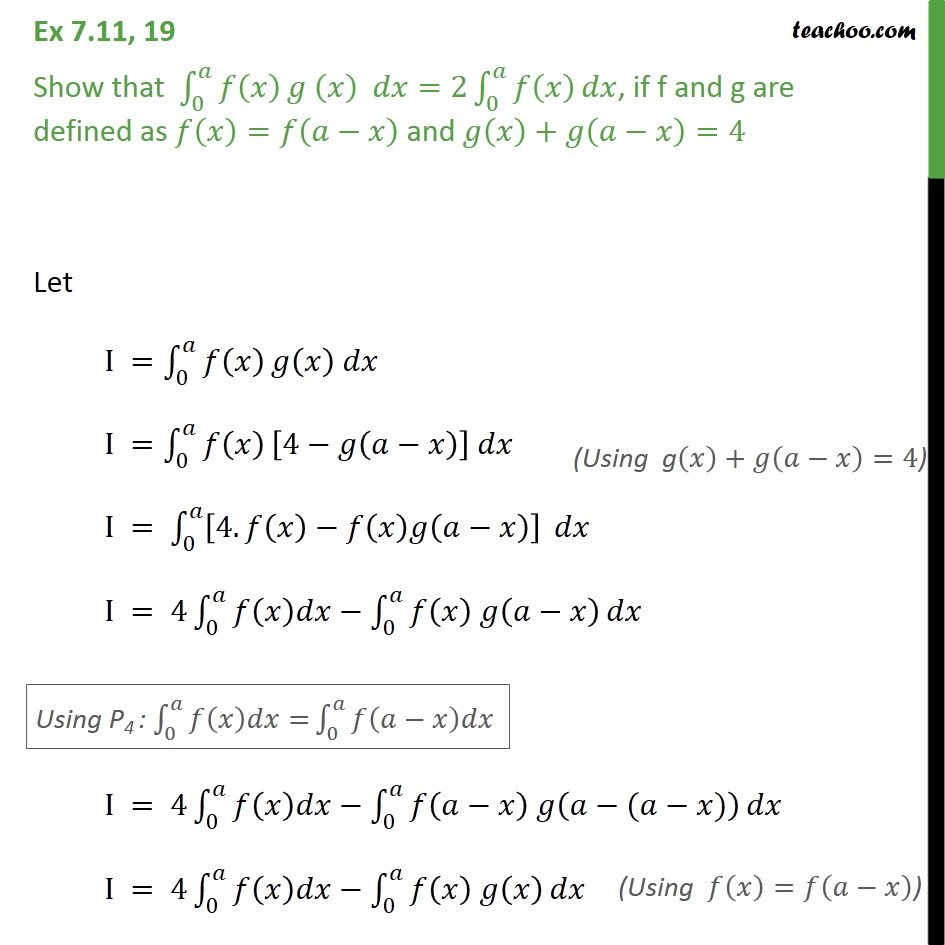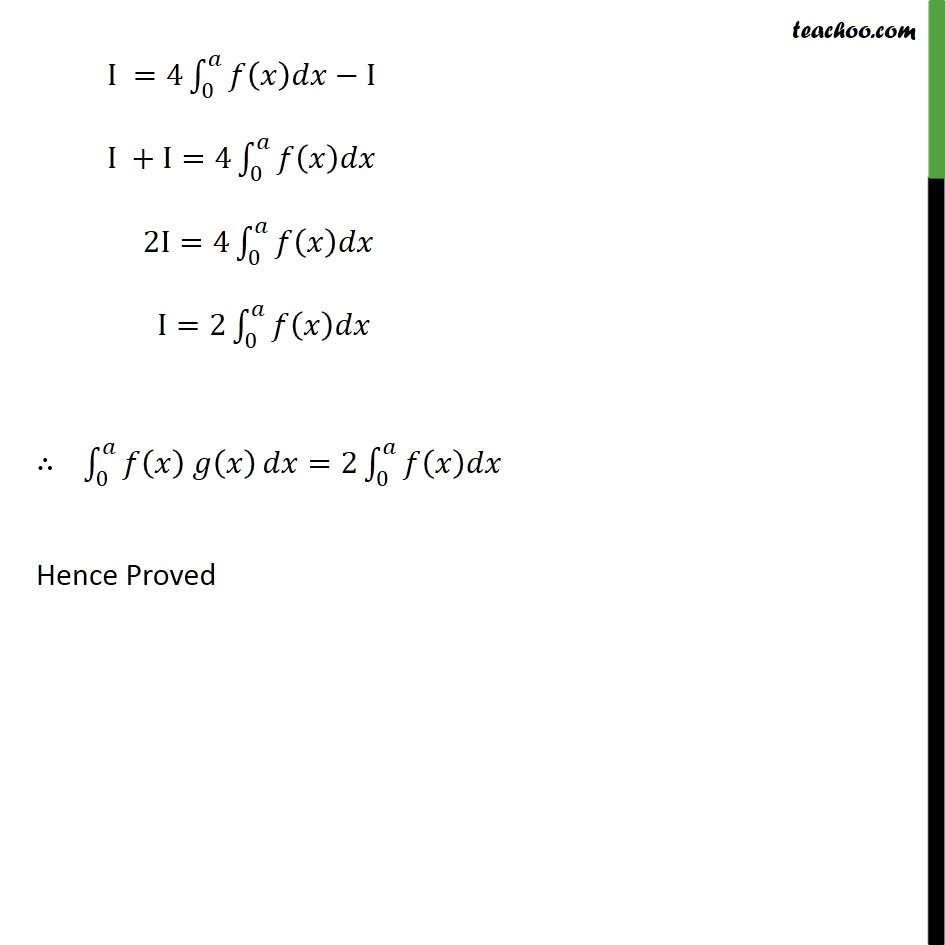Ex 7.10

Chapter 7 Class 12 Integrals
Serial order wiseLearn in your speed, with individual attention - Teachoo Maths 1-on-1 Class

### Transcript

Ex 7.10, 19 Show that _0^ ( ) ( ) =2 _0^ ( ) , if f and g are defined as ( )= ( ) and ( )+ ( )=4 Let I = _0^ ( ) ( ) I = _0^ ( ) [4 ( )] I = _0^ [4. ( ) ( ) ( )] I = 4 _0^ ( ) _0^ ( ) ( ) I = 4 _0^ ( ) _0^ ( ) ( ( )) I = 4 _0^ ( ) _0^ ( ) ( ) I =4 _0^ ( ) I I +I=4 _0^ ( ) 2I=4 _0^ ( ) I=2 _0^ ( ) _0^ ( ) ( ) =2 _0^ ( ) Hence Proved